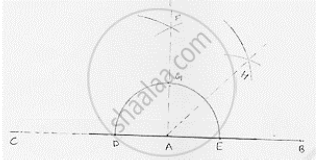# Construct the Following Angles at the Initial Point of a Given Ray and Justify the Construction 45° - Mathematics

Construct the following angles at the initial point of a given ray and justify the construction 45°

#### SolutionSteps of construction:
1. Draw a line segment AB and produce BA to point C.
2. With center A and any radius drawn an arc which intersect AC at D and AB at E.
3. With center D and E and radius more than 1/2DE draw arcs cutting each other at F.
4. Join FA which intersect arc in (2) at G.
5. With centers G and E and radius more than 1/2GE draw arcs cutting each other at H.
6. Join HA
∴∠HAB = 45°

Concept: Basic Constructions
Is there an error in this question or solution?

#### APPEARS IN

RD Sharma Mathematics for Class 9
Chapter 16 Constructions
Exercise 16.2 | Q 10.1 | Page 9

Share